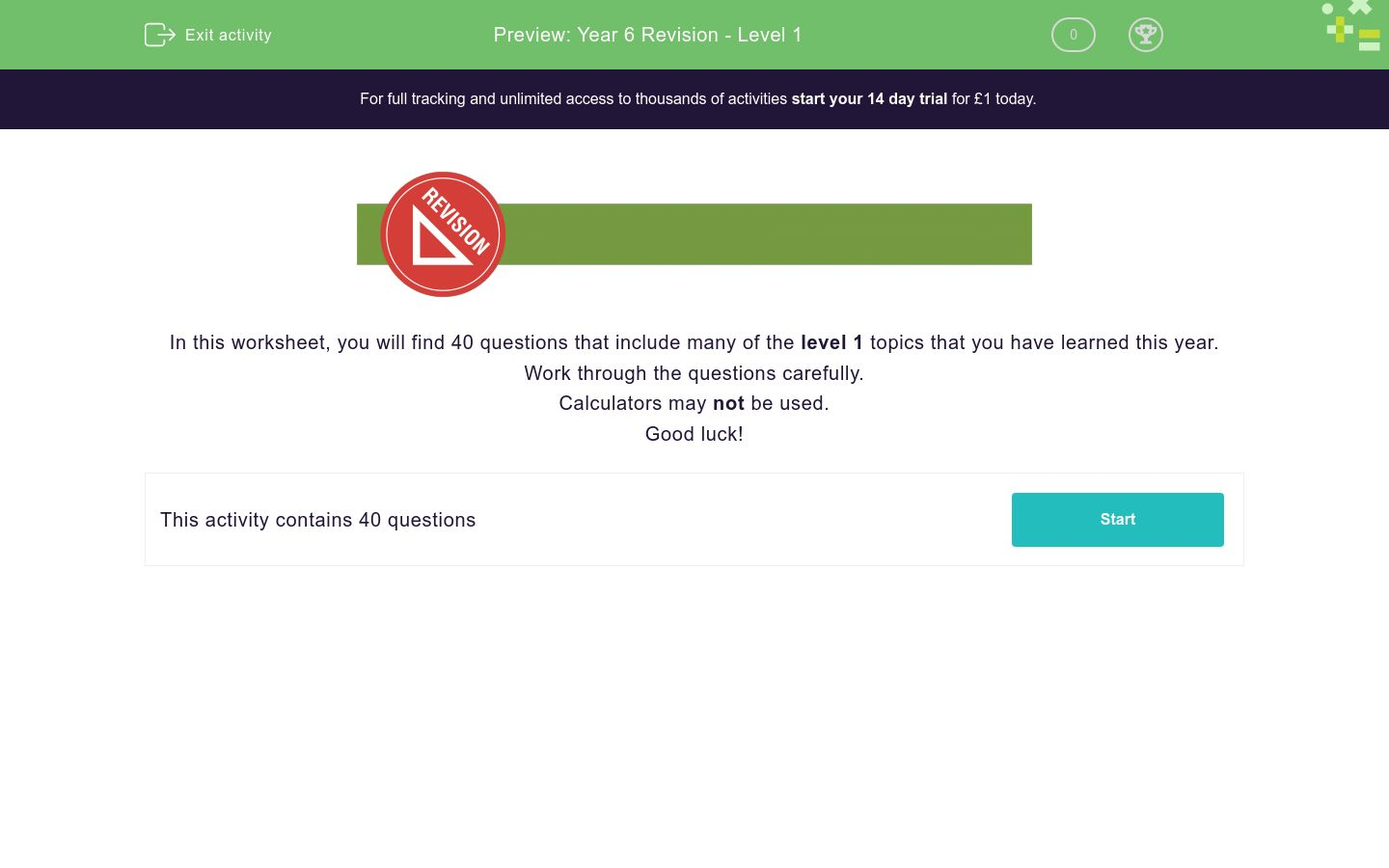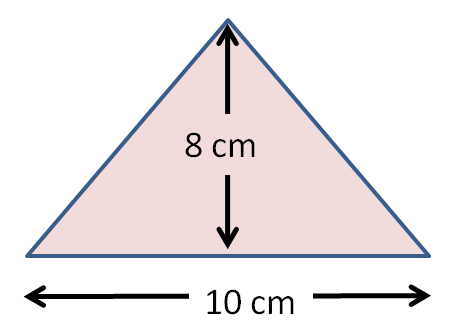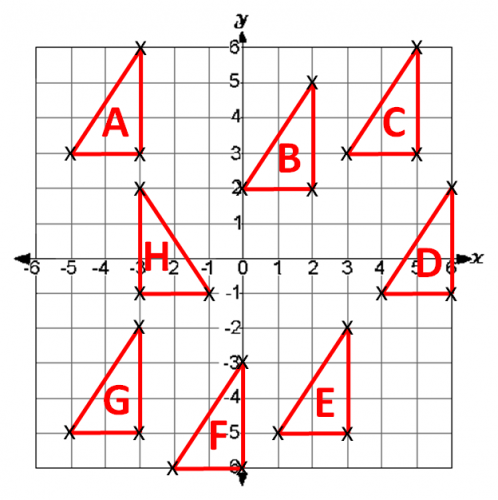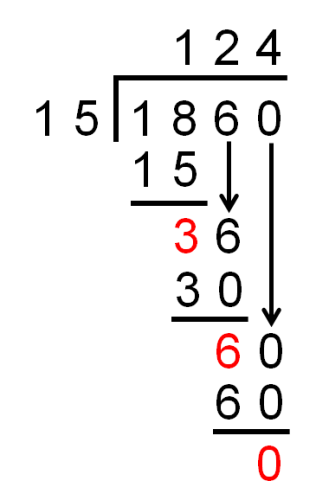# Year 6 Revision - Level 1

This is a level 1 revision exercise to help prepare for Year 6/end of Key Stage 2 tests.Key stage:  KS 2

Curriculum topic:   Revision

Curriculum subtopic:   Year 6 Revision

Difficulty level:### QUESTION 1 of 10In this worksheet, you will find 40 questions that include many of the level 1 topics that you have learned this year.

Work through the questions carefully.

Calculators may not be used.

Good luck!

Write this number using figures:

Fourteen thousand and twenty-twoWhen you write the following number using digits, select the column in which you would place the digit  3.

Four million, six hundred and thirty-eight thousand, seven hundred and ninety

Millions

Hundred Thousands

Ten Thousands

Thousands

Hundreds

Tens

Units

Compare:

521 625532 504

(Choose the correct symbol.)

<

=

>

Round to the nearest million:

5 456 011

5 000 000

6 000 000

Select all the prime numbers in this list.

1

2

3

4

5

9

13

25

29

33

34 + 26

Subtract:

4653 - 2846

(Use a piece of paper, and set the numbers out in a column subtraction.)

Use long multiplication to work out:

616 × 31

Use multiplication and division to work out the value of each letter in this multiplication grid.

x

A 8 F 5
8 24 64 56 40
E 12 C 28 D
7 21 B 49 35

## Column B

A
56
B
4
C
20
D
7
E
32
F
3

Use long division to work out:

1860 ÷ 15

Write down the number which is halfway between:

5400 and 10400

Work out:

7 → + 9× 2- 8÷ 4 → ?

Work out:

(15 + 3) ÷ 3

Choose the fraction which is equivalent to the decimal:

0.375

375/10

375/100

375/1000

375/1

Convert the following percentage to a decimal:

99%

The following improper fraction is changed to a mixed number:

 19 3

Which is the correct answer, A or B?

 A. 3 1 B. 6 1 6 3
A

B

Work out half of:

0.68

Work out:

2.683 + 5.1

Work out:

7.8 × 3

Work out:

7.8 ÷ 3

Look at the shading in this rectangle.1/5

1/2

2

Work out:

10% of 200

When we work out this calculation, what is the lowest common denominator we should choose for both fractions?

 3 + 5 8 6

Work out the following, remembering to give your answer in its lowest terms, e.g. 7/10.

 4 × 3 7 4

Look at these similar rectangles.

What is the ratio of their lengths, reduced to its lowest terms?5:10

1:2

3:6

10:5

There are 15 apples in a tray.

Ann and Bill share them out.

For every two that Ann gets, Bill gets one.

How many apples does Bill get?

I think of a number.

I double it and add 7.

I get 21.

Write this using algebra.

Use n for the number I think of.

7n + 2 = 21

7n - 2 = 21

2n + 7 = 21

2n - 7 = 21

A sequence starts at 15 and follows the rule to add 3 to the previous term.

What is the 4th term of this sequence?

Calculate the area of this triangle in cm2.

(Just write the number.)5 miles is the same distance as 8 kilometres.

How far is 35 miles in kilometres?

(Just write the number.)

Jack pours 3 litres of water into a pan and then removes 500 ml.

How much is left in the pan in litres?

(Just write the number.)

Look at this circle.a

b

c

d

Two of the angles in a triangle are 95º and  35º.

Work out the size of the third angle in degrees.

(Just write the number.)

Look at the coordinate grid below.

What are the coordinates of the point F?(5, -2)

(5, 2)

(-5, 2)

(2, -5)

(-2, 5)

On paper, draw a set of x-y coordinate axes like these.Follow these instructions to plot points and draw lines.

Name the shape that is formed.

Join (-2, -3) to (0, -3).

Join (0, -3) to (-1, -5).

Join (-1, -5) to (-2, -3).

isosceles triangle

right-angled triangle

square

rectangle

parallelogram

Look at the triangles on this coordinate grid.

On which triangle does Triangle A land when it is translated:

8 squares down.The children in a football team were asked to say their shoe size.

Work out the MODAL value or the MODE of this list.

5,  8,  7,  7,  6,  5,  6,  7,  6,  8,  7

This pie chart shows the results of a survey on favourite crisp flavours.

40 people took part in the survey.Look at this line graph, which shows the height of a tomato plant, measured each week.How tall was the plant in cm after 4 weeks?

(Just write the number.)

Amy has 22 marbles.

Ben has 25 marbles.

Charlie has 18 marbles.

They place all the marbles into a bowl and then each take one out of the bowl in turn, until it's no longer fair to do so.

How many are left in the bowl?

• Question 1

Write this number using figures:

Fourteen thousand and twenty-two

14022
14 022
EDDIE SAYS
Fourteen thousand would be 14 000.
There are no hundreds.
Then we need 22 in the tens and units columns.
• Question 2When you write the following number using digits, select the column in which you would place the digit  3.

Four million, six hundred and thirty-eight thousand, seven hundred and ninety

Ten Thousands
EDDIE SAYS
38 thousand is thirty thousand and eight thousand.
Thirty thousand is shown by a 3 in the ten thousands column.
• Question 3

Compare:

521 625532 504

(Choose the correct symbol.)

<
EDDIE SAYS
Compare the 10 thousands, as the 100 thousands are the same.
• Question 4

Round to the nearest million:

5 456 011

5 000 000
EDDIE SAYS
The 4 in the hundred thousands place tells you to round down to 5 million.
• Question 5

Select all the prime numbers in this list.

2
3
5
13
29
EDDIE SAYS
Prime numbers only have two factors - the number itself and 1.
• Question 6

34 + 26

60
EDDIE SAYS
30 + 20 = 50
4 + 6 = 10
Add together 50 + 10 = 60.
• Question 7

Subtract:

4653 - 2846

(Use a piece of paper, and set the numbers out in a column subtraction.)

1807
EDDIE SAYS• Question 8

Use long multiplication to work out:

616 × 31

19096
19 096
EDDIE SAYS
616 × 1 = 616
616 × 30 = 18 480
• Question 9

Use multiplication and division to work out the value of each letter in this multiplication grid.

x

A 8 F 5
8 24 64 56 40
E 12 C 28 D
7 21 B 49 35

## Column B

A
3
B
56
C
32
D
20
E
4
F
7
EDDIE SAYS
A = 24 ÷ 8
B = 7 × 8
E = 12 ÷ A = 12 ÷ 3 = 4
C = E × 8 = 4 × 8 = 32
D = E × 5 = 4 × 5 = 20
F = 56 ÷ 8
• Question 10

Use long division to work out:

1860 ÷ 15

124
EDDIE SAYS• Question 11

Write down the number which is halfway between:

5400 and 10400

7900
EDDIE SAYS
Difference is 5000.
Halve this to get 2500.
• Question 12

Work out:

7 → + 9× 2- 8÷ 4 → ?

6
EDDIE SAYS
7 → 16 → 32 → 24 → 6
• Question 13

Work out:

(15 + 3) ÷ 3

6
EDDIE SAYS
Work out the brackets first: 15 + 3 = 18.
18 ÷ 3 = 6
• Question 14

Choose the fraction which is equivalent to the decimal:

0.375

375/1000
EDDIE SAYS
Three decimal places means thousandths.
• Question 15

Convert the following percentage to a decimal:

99%

0.99
EDDIE SAYS
99 ÷ 100 = 0.99
• Question 16

The following improper fraction is changed to a mixed number:

 19 3

Which is the correct answer, A or B?

 A. 3 1 B. 6 1 6 3
B
EDDIE SAYS
19 ÷ 3 = 6 remainder 1
• Question 17

Work out half of:

0.68

0.34
EDDIE SAYS
68 ÷ 2 = 34
0.68 ÷ 2 = 0.34
• Question 18

Work out:

2.683 + 5.1

7.783
EDDIE SAYS
Add units to units and tenths to tenths.
• Question 19

Work out:

7.8 × 3

23.4
EDDIE SAYS
78 × 3 = 234
7.8 × 3 = 23.4
• Question 20

Work out:

7.8 ÷ 3

2.6
EDDIE SAYS
78 ÷ 3 = 26
7.8 ÷ 3 = 2.6
• Question 21

Look at the shading in this rectangle.1/5
EDDIE SAYS
1/5 is the same as 2/10.
• Question 22

Work out:

10% of 200

20
EDDIE SAYS
1% of 200 is 200 ÷ 100 = 2.
10% is 10 × 2 = 20.
• Question 23

When we work out this calculation, what is the lowest common denominator we should choose for both fractions?

 3 + 5 8 6
24
EDDIE SAYS
The lowest common multiple of 8 and 6 is 24.
• Question 24

Work out the following, remembering to give your answer in its lowest terms, e.g. 7/10.

 4 × 3 7 4

3/7
EDDIE SAYS
4 × 3 = 12
7 × 4 = 28
12/28 reduces to 3/7 by dividing top and bottom by 4.
• Question 25

Look at these similar rectangles.

What is the ratio of their lengths, reduced to its lowest terms?1:2
EDDIE SAYS
3:6 and 5:10 reduce like a fraction to 1:2.
• Question 26

There are 15 apples in a tray.

Ann and Bill share them out.

For every two that Ann gets, Bill gets one.

How many apples does Bill get?

5
EDDIE SAYS
They do this 15 ÷ 3 = 5 times.
Bill gets 5 × 1 = 5 apples.
• Question 27

I think of a number.

I double it and add 7.

I get 21.

Write this using algebra.

Use n for the number I think of.

2n + 7 = 21
EDDIE SAYS
Double n and add 7 to get 21.
• Question 28

A sequence starts at 15 and follows the rule to add 3 to the previous term.

What is the 4th term of this sequence?

24
EDDIE SAYS
The sequence is 15, 18, 21, 24, 27, ...
• Question 29

Calculate the area of this triangle in cm2.

(Just write the number.)40
EDDIE SAYS
Base length = 10 cm
Perpendicular height = 8 cm
Area = 10 × 8 ÷ 2 = 80 ÷ 2 = 40 cm2
• Question 30

5 miles is the same distance as 8 kilometres.

How far is 35 miles in kilometres?

(Just write the number.)

56
EDDIE SAYS
35 ÷ 5 = 7
7 × 8 = 56 km
• Question 31

Jack pours 3 litres of water into a pan and then removes 500 ml.

How much is left in the pan in litres?

(Just write the number.)

2.5
EDDIE SAYS
3000 ml - 500 ml = 2500 ml
2500 ÷ 1000 = 2.5 l
• Question 32

Look at this circle.b
EDDIE SAYS
The radius goes from the centre to the circumference.
• Question 33

Two of the angles in a triangle are 95º and  35º.

Work out the size of the third angle in degrees.

(Just write the number.)

50
EDDIE SAYS
The angles will all add up to 180°.
95 + 35 = 130
180 - 130 = 50
• Question 34

Look at the coordinate grid below.

What are the coordinates of the point F?(-5, 2)
EDDIE SAYS
-5 on the x-axis.
2 on the y-axis.
• Question 35

On paper, draw a set of x-y coordinate axes like these.Follow these instructions to plot points and draw lines.

Name the shape that is formed.

Join (-2, -3) to (0, -3).

Join (0, -3) to (-1, -5).

Join (-1, -5) to (-2, -3).

isosceles triangle
EDDIE SAYS• Question 36

Look at the triangles on this coordinate grid.

On which triangle does Triangle A land when it is translated:

8 squares down.G
EDDIE SAYS
Each corner of Triangle A moves 8 units down and lands on Triangle G.
• Question 37

The children in a football team were asked to say their shoe size.

Work out the MODAL value or the MODE of this list.

5,  8,  7,  7,  6,  5,  6,  7,  6,  8,  7

7
EDDIE SAYS
7 is the one that is listed the most times.
• Question 38

This pie chart shows the results of a survey on favourite crisp flavours.

40 people took part in the survey.10
EDDIE SAYS
¼ of the group chose Ready Salted.
40 ÷ 4 = 10 people.
• Question 39

Look at this line graph, which shows the height of a tomato plant, measured each week.How tall was the plant in cm after 4 weeks?

(Just write the number.)

20
21
EDDIE SAYS
From 4 on the Weeks axis, go up to the graph line, then left to the Height axis.
• Question 40

Amy has 22 marbles.

Ben has 25 marbles.

Charlie has 18 marbles.

They place all the marbles into a bowl and then each take one out of the bowl in turn, until it's no longer fair to do so.

How many are left in the bowl?

2
EDDIE SAYS
22 + 25 + 18 = 65 marbles.
65 ÷ 3 = 21 r2
They get 21 each and 2 are left in the bowl.
---- OR ----

Sign up for a £1 trial so you can track and measure your child's progress on this activity.

### What is EdPlace?

We're your National Curriculum aligned online education content provider helping each child succeed in English, maths and science from year 1 to GCSE. With an EdPlace account you’ll be able to track and measure progress, helping each child achieve their best. We build confidence and attainment by personalising each child’s learning at a level that suits them.

Get started